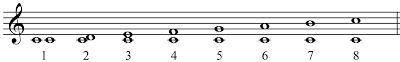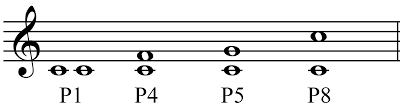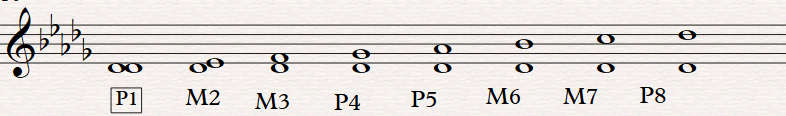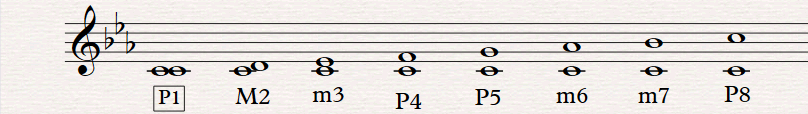# Introduction To Intervals In Music

In music theory, an interval?is the distance in pitch between two notes.
To identify an interval you will need two things: a number and a type (quality).

## List of interval (number):

• Unison ? 1st
• Second – 2nd
• Third – 3rd
• Fourth – 4th
• Fifth – 5th
• Sixth – 6th
• Seventh – 7th
• Octave ? 8ve
• Ninth – 9th
• etc.

Each interval will have a number – 1, 2, 3, etc., based upon counting the?lines and spaces?on the staff.For example, if we count lines and spaces, starting from C and ending on E, we count: C,D,E = 1,2,3. Therefore, the interval from C to E is a third (3rd). Or if we count lines and spaces, starting from E and ending on A above, we count: E,F,G,A = 1,2,3,4. Therefore, the interval from E to A is a fourth (4th).

We can also keep counting past 8, through 9, 10, 11, etc, but in this case we have the option to identify them as compound intervals. For example, the interval from C to D being a ninth (9th) can also be identified as a compound 2nd.

Interval type (quality):
Intervals are also identified by their type. Intervals can be called Major (M), minor (m), Perfect (P), Augmented (A), or diminished (d).

If we look at the intervals produced in a major scale, comparing each degree of the scale to the key-note (tonic), here is the complete list of the intervals:

## Major Intervals## Perfect Intervals:The same intervals are therefore produced for any major key.
For example, for Db Major scale:For this reason, to have a good understanding of the intervals and how to identify them, it is very important to know the scales.

If we look at the intervals produced from a key-note of a natural minor scale, we will notice that the only intervals which are different from those of the major scale with the same tonic, will be the 3rd, the 6th and the 7th. Here are the intervals for C minor:These new intervals, which have a semitone less than the correspondent major interval, are called respectively 3rd, 6th and 7th minor.

As mentioned above there are other intervals, called augmented and diminished. These intervals can be produced as follows:

Augmented, by raising a semitone the upper note of a perfect or major interval. For example C-G is a perfect 5th, so C-G# is an augmented 5th.

Diminished, by lowering a semitone the upper note of a perfect or minor chord. For example C-Gb is a diminished 5th.

Each interval comprises of a certain number of?semitones. With the aid of a?piano?it is easy to count the number of semitones that make up intervals.

Remember: even if the knowledge of scales is limited, it is enough to remember that C major and A natural minor scales both only use white keys, to be able to work out the list below without need to memorise it.

## List of intervals:

• P1, d2 = 0 semitones
• m2, A1 = 1 semitone
• M2, d3 = 2 semitones
• m3, A2 = 3 semitones
• M3, d4 = 4 semitones
• P4, A3 = 5 semitones
• A4, d5 = 6 semitones
• P5, d6 = 7 semitones
• m6, A5 = 8 semitones
• M6, d7 = 9 semitones
• m7, A6 = 10 semitones
• M7, d8 = 11 semitones
• P8, A7 = 12 semitones

It is good to be aware of how many semitones are needed to make an interval, so that answers can be checked: for example, if we believe that D-Ab is a diminished 5th, we can test our answers by counting the number of semitones (6), which in this case will confirm that our answer is correct.

Finally, it should be noted that for each number the various types are all one semitone apart, diminished being the smallest and augmented the largest.

2nd /3rd /6th /7th Intervals can be Diminished/Minor/Major/Augmented

Unison/4th / 5th / 8ve Intervals can be Diminished/Perfect/Augmented

For example, C-E is a major 3rd (4 semitones): therefore an augmented 3rd will have 5 semitones (C-E#), a minor 3rd of 3 (C-Bb) and a diminished 3rd 2 (C-Bbb).

Another example: C-G is a perfect 5th (7 semitones), therefore an augmented 5th will have 8 semitones and a diminished 5th 6.